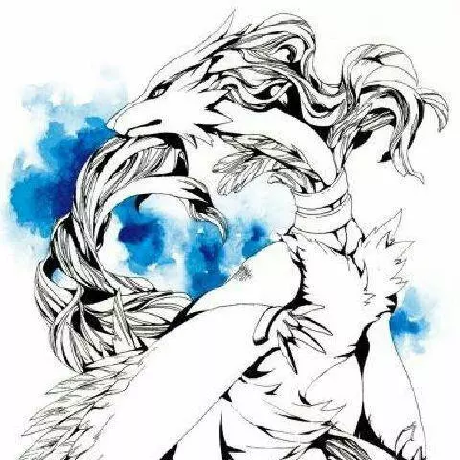+关注继续查看

# 标注

## 基本标注

import numpy as np
import matplotlib.pyplot as plt

fig = plt.figure()

t = np.arange(0.0, 5.0, 0.01)
s = np.cos(2*np.pi*t)
line, = ax.plot(t, s, lw=2)

ax.annotate('local max', xy=(2, 1), xytext=(3, 1.5),
arrowprops=dict(facecolor='black', shrink=0.05),
)

ax.set_ylim(-2,2)
plt.show()| 参数 | 坐标系 |
| 'figure points' | 距离图形左下角的点数量 |
| 'figure pixels' | 距离图形左下角的像素数量 |
| 'figure fraction' | 0,0 是图形左下角，1,1 是右上角 |
| 'axes points' | 距离轴域左下角的点数量 |
| 'axes pixels' | 距离轴域左下角的像素数量 |
| 'axes fraction' | 0,0 是轴域左下角，1,1 是右上角 |
| 'data' | 使用轴域数据坐标系 |

ax.annotate('local max', xy=(3, 1),  xycoords='data',
xytext=(0.8, 0.95), textcoords='axes fraction',
arrowprops=dict(facecolor='black', shrink=0.05),
horizontalalignment='right', verticalalignment='top',
)

arrowprops 描述
width 箭头宽度，以点为单位
frac 箭头头部所占据的比例
headwidth 箭头的底部的宽度，以点为单位
shrink 移动提示，并使其离注释点和文本一些距离
**kwargs matplotlib.patches.Polygon的任何键，例如facecolor## 高级标注

### 使用框和文本来标注pyplot模块（或Axes类的text方法）中的text()函数接受bbox关键字参数，并且在提供时，在文本周围绘制一个框。

bb = t.get_bbox_patch()

bb.set_boxstyle("rarrow", pad=0.6)

Circle circle pad=0.3
DArrow darrow pad=0.3
LArrow larrow pad=0.3
RArrow rarrow pad=0.3
Round round pad=0.3,rounding_size=None
Round4 round4 pad=0.3,rounding_size=None
Roundtooth roundtooth pad=0.3,tooth_size=None
Sawtooth sawtooth pad=0.3,tooth_size=None
Square square pad=0.3bb.set_boxstyle("rarrow,pad=0.6")

### 使用箭头来标注

pyplot模块（或Axes类的annotate方法）中的annotate()函数用于绘制连接图上两点的箭头。

ax.annotate("Annotation",
xy=(x1, y1), xycoords='data',
xytext=(x2, y2), textcoords='offset points',
)

ax.annotate("",
xy=(0.2, 0.2), xycoords='data',
xytext=(0.8, 0.8), textcoords='data',
arrowprops=dict(arrowstyle="->",
connectionstyle="arc3"),
)• 创建两个点之间的连接路径。 这由connectionstyle键值控制。
• 如果提供了补丁对象（patchApatchB），则会剪切路径以避开该补丁。
• 路径进一步由提供的像素总量来缩小（shirnkA&shrinkB
• 路径转换为箭头补丁，由arrowstyle键值控制。angle angleA=90,angleB=0,rad=0.0
angle3 angleA=90,angleB=0
arc angleA=0,angleB=0,armA=None,armB=None,rad=0.0
arc3 rad=0.0
bar armA=0.0,armB=0.0,fraction=0.3,angle=None- None
-> head_length=0.4,head_width=0.2
-[ widthB=1.0,lengthB=0.2,angleB=None
|-| widthA=1.0,widthB=1.0
-|> head_length=0.4,head_width=0.2
<- head_length=0.4,head_width=0.2
<-> head_length=0.4,head_width=0.2
<|- head_length=0.4,head_width=0.2
< -|>
fancy head_length=0.4,head_width=0.4,tail_width=0.4
simple head_length=0.5,head_width=0.5,tail_width=0.2
wedge tail_width=0.3,shrink_factor=0.5text命令一样，可以使用bbox参数来绘制文本周围的框。### 将艺术家放置在轴域的锚定位置

from mpl_toolkits.axes_grid.anchored_artists import AnchoredText
at = AnchoredText("Figure 1a",
prop=dict(size=8), frameon=True,
loc=2,
)
ax.add_artist(at)loc关键字与legend命令中含义相同。

from mpl_toolkits.axes_grid.anchored_artists import AnchoredDrawingArea

ada = AnchoredDrawingArea(20, 20, 0, 0,
p1 = Circle((10, 10), 10)
p2 = Circle((30, 10), 5, fc="r")
ada.drawing_area.add_artist(p2)from mpl_toolkits.axes_grid.anchored_artists import AnchoredAuxTransformBox

box = AnchoredAuxTransformBox(ax.transData, loc=2)
el = Ellipse((0,0), width=0.1, height=0.4, angle=30) # in data coordinates!
box.drawing_area.add_artist(el)### 使用复杂坐标来标注

matplotlib 中的标注支持标注文本中描述的几种类型的坐标。 对于想要更多控制的高级用户，它支持几个其他选项。

1. Transform实例，例如：

ax.annotate("Test", xy=(0.5, 0.5), xycoords=ax.transAxes)

相当于：

ax.annotate("Test", xy=(0.5, 0.5), xycoords="axes fraction")

使用它，你可以在其他轴域内标注一个点：

ax1, ax2 = subplot(121), subplot(122)
ax2.annotate("Test", xy=(0.5, 0.5), xycoords=ax1.transData,
xytext=(0.5, 0.5), textcoords=ax2.transData,
arrowprops=dict(arrowstyle="->"))
2. Artist实例。xy值（或xytext）被解释为艺术家的bboxget_window_extent的返回值）的小数坐标。

an1 = ax.annotate("Test 1", xy=(0.5, 0.5), xycoords="data",
va="center", ha="center",
bbox=dict(boxstyle="round", fc="w"))
an2 = ax.annotate("Test 2", xy=(1, 0.5), xycoords=an1, # (1,0.5) of the an1's bbox
xytext=(30,0), textcoords="offset points",
va="center", ha="left",
bbox=dict(boxstyle="round", fc="w"),
arrowprops=dict(arrowstyle="->"))

源代码请注意，你的责任是在绘制an2之前确定坐标艺术家（上例中的an1）的范围。 在大多数情况下，这意味着an2需要晚于an1

3. 一个返回BboxBaseTransform的实例的可调用对象。 如果返回一个变换，它与 1 相同，如果返回bbox，它与 2 相同。可调用对象应该接受renderer实例的单个参数。 例如，以下两个命令产生相同的结果：

an2 = ax.annotate("Test 2", xy=(1, 0.5), xycoords=an1,
xytext=(30,0), textcoords="offset points")
an2 = ax.annotate("Test 2", xy=(1, 0.5), xycoords=an1.get_window_extent,
xytext=(30,0), textcoords="offset points")
4. 指定二元坐标的元组。 第一项用于x坐标，第二项用于y坐标。 例如，

annotate("Test", xy=(0.5, 1), xycoords=("data", "axes fraction"))

0.5 的单位是数据坐标，1 的单位是归一化轴域坐标。 你可以像使用元组一样使用艺术家或变换。 例如，

import matplotlib.pyplot as plt

plt.figure(figsize=(3,2))
ax=plt.axes([0.1, 0.1, 0.8, 0.7])
an1 = ax.annotate("Test 1", xy=(0.5, 0.5), xycoords="data",
va="center", ha="center",
bbox=dict(boxstyle="round", fc="w"))

an2 = ax.annotate("Test 2", xy=(0.5, 1.), xycoords=an1,
xytext=(0.5,1.1), textcoords=(an1, "axes fraction"),
va="bottom", ha="center",
bbox=dict(boxstyle="round", fc="w"),
arrowprops=dict(arrowstyle="->"))
plt.show()

源代码5. 有时，您希望您的注释带有一些“偏移点”，不是距离注释点，而是距离某些其他点。 OffsetFrom是这种情况下的辅助类。

import matplotlib.pyplot as plt

plt.figure(figsize=(3,2))
ax=plt.axes([0.1, 0.1, 0.8, 0.7])
an1 = ax.annotate("Test 1", xy=(0.5, 0.5), xycoords="data",
va="center", ha="center",
bbox=dict(boxstyle="round", fc="w"))

from matplotlib.text import OffsetFrom
offset_from = OffsetFrom(an1, (0.5, 0))
an2 = ax.annotate("Test 2", xy=(0.1, 0.1), xycoords="data",
xytext=(0, -10), textcoords=offset_from,
# xytext is offset points from "xy=(0.5, 0), xycoords=an1"
va="top", ha="center",
bbox=dict(boxstyle="round", fc="w"),
arrowprops=dict(arrowstyle="->"))
plt.show()### 使用ConnectorPatch

ConnectorPatch类似于没有文本的标注。 虽然在大多数情况下建议使用标注函数，但是当您想在不同的轴上连接点时，ConnectorPatch很有用。

from matplotlib.patches import ConnectionPatch
xy = (0.2, 0.2)
con = ConnectionPatch(xyA=xy, xyB=xy, coordsA="data", coordsB="data",
axesA=ax1, axesB=ax2)
ax2.add_artist(con)## 高级话题

### 轴域之间的缩放效果

mpl_toolkits.axes_grid.inset_locator定义了一些补丁类，用于互连两个轴域。 理解代码需要一些 mpl 转换如何工作的知识。 但是，利用它的方式很直接。### 定义自定义盒样式

def __call__(self, x0, y0, width, height, mutation_size,
aspect_ratio=1.):
"""
Given the location and size of the box, return the path of
the box around it.

- *x0*, *y0*, *width*, *height* : location and size of the box
- *mutation_size* : a reference scale for the mutation.
- *aspect_ratio* : aspect-ratio for the mutation.
"""
path = ...
return pathfrom matplotlib.path import Path
from matplotlib.patches import BoxStyle
import matplotlib.pyplot as plt

# we may derive from matplotlib.patches.BoxStyle._Base class.
# You need to override transmute method in this case.

class MyStyle(BoxStyle._Base):
"""
A simple box.
"""

"""
The arguments need to be floating numbers and need to have
default values.

"""

super(MyStyle, self).__init__()

def transmute(self, x0, y0, width, height, mutation_size):
"""
Given the location and size of the box, return the path of
the box around it.

- *x0*, *y0*, *width*, *height* : location and size of the box
- *mutation_size* : a reference scale for the mutation.

Often, the *mutation_size* is the font size of the text.
You don't need to worry about the rotation as it is
automatically taken care of.
"""

width, height = width + 2.*pad, \

# boundary of the padded box
x1, y1 = x0+width, y0 + height

cp = [(x0, y0),
(x1, y0), (x1, y1), (x0, y1),
(x0, y0)]

com = [Path.MOVETO,
Path.LINETO, Path.LINETO, Path.LINETO,
Path.LINETO, Path.LINETO,
Path.CLOSEPOLY]

path = Path(cp, com)

return path

# register the custom style
BoxStyle._style_list["angled"] = MyStyle

plt.figure(1, figsize=(3,3))
ax = plt.subplot(111)
ax.text(0.5, 0.5, "Test", size=30, va="center", ha="center", rotation=30,

del BoxStyle._style_list["angled"]

plt.show()Matplotlib 中文用户指南 8.2 我们最喜欢的秘籍

711 0Matplotlib 中文用户指南 8.1 屏幕截图

1102 0Matplotlib 中文用户指南 7.3 事件处理及拾取

1107 0Matplotlib 中文用户指南 7.2 Python shell 中使用 Matplotlib

889 0Matplotlib 中文用户指南 7.1 交互式导航

615 0Matplotlib 中文用户指南 6 自定义 matplotlib

516 0Matplotlib 中文用户指南 5.1 指定颜色

488 0Matplotlib 中文用户指南 4.8 XeLaTeX/LuaLaTeX 设置
XeLaTeX/LuaLaTeX 设置 原文：Typesetting With XeLaTeX/LuaLaTeX 译者：飞龙 协议：CC BY-NC-SA 4.0 使用 pgf 后端，matplotlib 可以将图形导出为可以使用 pdflatex，xelatex 或 lualatex 处理的 pgf 绘图命令。
946 0Matplotlib 中文用户指南 4.7 使用 LaTeX 渲染文本

887 0Matplotlib 中文用户指南 4.6 编写数学表达式

878 0
apachecn_飞龙
Github：@wizardforcel 简书：@ApacheCN_飞龙 微博：@龙雀 CSDN：@wizardforcel ApacheCN 官网：apachecn.org 机器学习交流群：629470233

【视觉与图像】Python+OpenCV教程入门篇-1月16日更新7816

Python 数据可视化库 Matplotlib 快速入门4080518

【视觉与图像】Python+OpenCV教程基础篇8514